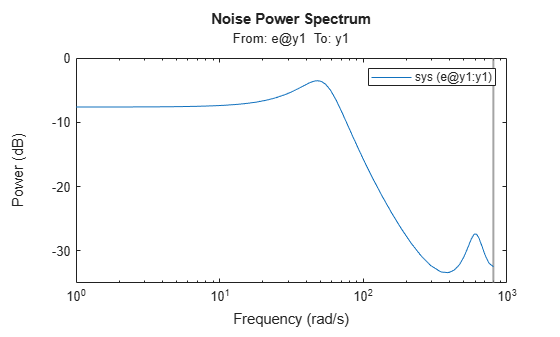Main Content

# spectrum

Plot or return output power spectrum of time series model or disturbance spectrum of linear input-output model

## Syntax

``spectrum(sys)``
``spectrum(sys,{wmin, wmax})``
``spectrum(sys,w)``
``spectrum(sys1,...,sysN,w)``
``ps = spectrum(sys,w)``
``ps = spectrum(sys,w)``
``[ps,wout] = spectrum(sys)``
``````[ps,wout,sdps] = spectrum(sys)``````

## Description

### Plot Results

example

````spectrum(sys)` plots the output power spectrum of an identified time series model `sys` or the disturbance spectrum of an identified input-output model `sys`. The function chooses the frequency range and number of points automatically. If `sys` is a time series model, then `sys` represents the system:$y\left(t\right)=He\left(t\right)$Here, e(t) is a Gaussian white noise and y(t) is the observed output.`spectrum` plots |H'H|, scaled by the variance of e(t) and the sample time.If `sys` is an input-output model, `sys` represents the system:$y\left(t\right)=Gu\left(t\right)+He\left(t\right)$Here, u(t) is the measured input, e(t) is Gaussian white noise and y(t) is the observed output.In this case, `spectrum` plots the spectrum of the disturbance component He(t).For discrete-time models with sample time Ts, `spectrum` uses the transformation $z={e}^{j\omega {T}_{s}}$ to map the unit circle to the real frequency axis. The function plots the spectrum only for frequencies smaller than the Nyquist frequency π/Ts, and uses the default value of 1 time unit when Ts is unspecified.```

example

````spectrum(sys,{wmin, wmax})` creates a spectrum plot for frequencies ranging from `wmin` to `wmax`.```
````spectrum(sys,w)` creates a spectrum plot using the frequencies specified in the vector `w`.```

example

````spectrum(sys1,...,sysN,w)` creates a spectrum plot of several identified models on a single plot. The `w` argument is optional.You can specify a color, line style and marker for each model. For example, `spectrum(sys1,'r',sys2,'y--',sys3,'gx')` uses red for `sys1`, yellow dash markers for `sys2`, and green `x` markers for `sys3`.```

### Return Results

````ps = spectrum(sys,w)` returns the power spectrum amplitude of `sys` for the specified frequencies, `w`. There is no plot display.```
````ps = spectrum(sys,w)` returns the power spectrum amplitude of `sys` for the specified frequencies, `w`.```
````[ps,wout] = spectrum(sys)` returns the frequency vector, `wout`, for which the output power spectrum is computed.```
``````[ps,wout,sdps] = spectrum(sys)``` returns the estimated standard deviations of the power spectrum.```

## Examples

collapse all

Load the time-series estimation data.

`load iddata9 z9`

Estimate a fourth-order AR model using a least-squares approach.

`sys = ar(z9,4,'ls');`

Plot the output spectrum of the model.

`spectrum(sys);`Load the estimation data.

`load iddata1 z1;`

Estimate a single-input single-output state-space model.

`sys = n4sid(z1,2);`

Plot the noise spectrum for the model. Specify a frequency range from 0.1 to 50 rad/sec.

`spectrum(sys,{0.1,50});`The function plots the spectrum, but limits the frequency range to the Nyquist frequency of approximately 31.4 rad/sec.

Create an input consisting of the sum of five sinusoids, each spread over the full frequency range. Compare the spectrum of this signal with that of its square.

Create a sum-of-sinusoids input that extends for 20 periods, with each period containing 100 samples. Specify that the signal combine 5 sinusoids of random phase, using 10 trials to find the set with the lowest signal spread. For more information on this step, see `idinput`.

`u = idinput([100 1 20],'sine',[],[],[5 10 1]);`

Create an input-only `iddata` object `u` that contains the input u and has a period of 100.

`u = iddata([],u,1,'per',100);`

Square the input values and store them in new `iddata` object `u2.`

```u2 = u.u.^2; u2 = iddata([],u2,1,'per',100);```

Use `etfe` to estimate empirical transfer function models from `u` and `u2`. Plot the power spectra of these models together. Use different marker colors and types to distinguish the spectrum sources.

`spectrum(etfe(u),'r*',etfe(u2),'+')`The plot shows some frequency splitting where the `u2`-based spectrum does not line up with the `u1-`based spectrum, but instead, contains two spectral points that flank certain `u1`-based points. This indicates the nonlinearity of the squared system.

## Input Arguments

collapse all

Identified model, specified as an `idpoly` object, an `idproc` object, an `idss` object, or an `idtf` object.

• If `sys` is a time series model, then `sys` represents the system:

`$y\left(t\right)=He\left(t\right)$`

Here, `e(t)` is a Gaussian white noise and `y(t)` is the observed output.

• If `sys` is an input-output model, then `sys` represents the system:

`$y\left(t\right)=Gu\left(t\right)+He\left(t\right)$`

Here, `u(t)` is the measured input, `e(t)` is a Gaussian white noise and `y(t)` is the observed output.

Minimum frequency of the frequency range for which to plot the spectrum, specified as a positive number.

Specify `wmin` in `rad/TimeUnit`, where `TimeUnit` is `sys.TimeUnit`.

Maximum frequency of the frequency range for which to plot the spectrum, specified as a positive number. By default, the function uses the Nyquist frequency of `sys` as `wmax`.

Specify `wmax` in `rad/TimeUnit`, where `TimeUnit` is `sys.TimeUnit`. If you specify `wmax` to be greater than the Nyquist frequency, then `spectrum` will use the Nyquist frequency instead.

Frequencies for which to plot the spectrum, specified as a vector of positive numbers.

Specify `w` in `rad/TimeUnit`, where `TimeUnit` is `sys.TimeUnit`.

## Output Arguments

collapse all

Power spectrum amplitude returned as a numeric vector or a numeric array.

• For single-output models, `ps` is a 1-by-1- Nw array, where Nw is the length of the frequency vector.

• For multiple-output models, `ps` is an Ny-byNy-byNw array, where Ny is the number of outputs. `ps(:,:,k)` corresponds to the power spectrum for the frequency at `w(k)`.

For amplitude values in dB, type ```psdb = 10*log10(ps)```.

Frequencies for which the spectrum is plotted, returned as a numeric vector. If you supply `w` as an input argument, the function returns the identical vector in `wout`.

Estimated standard deviation of the power spectrum, returned as an array with the same dimensions as `ps`.

Introduced in R2012a

## SupportGet trial now